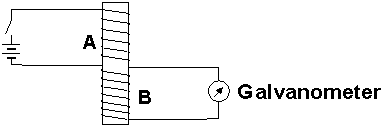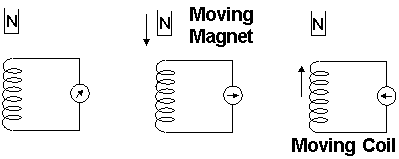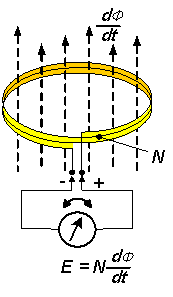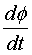# Moving Coils and Electromagnetic Induction

Electromagnetism > Moving Coils and Electromagnetic Induction

Michael Faraday (1791 – 1867) was an English chemist and physicist who is best known for his pioneering experiments in Electricity and Magnetism.

In 1831, Faraday made his discovery of Electromagnetic Induction, which is the production of an electrical potential difference across a conductor by changing the Magnetic Field near it. In his experiment, he wound two Coils on a wooden core, Figure 1. Every time he connected and disconnected the battery, the Galvanometer connecting Coil B deflects momentarily and returns to its original position.Figure 1: Faradays experiment on Induction

The momentary deflection is not a coincident at all. It is caused by the instant flow of Current in Coil B, which is induced due to a change in Magnetic Field in Coil A. The Magnetic Field change occurs whenever the battery is connected or disconnected.

Once Faraday had realized that change in a Magnetic Field could induce a Current in the nearby circuit, he substituted Coil A with a piece of Magnet, Figure 2. Either moving continuously the Coil or the Magnet induced a Current in the Coil. Aside from an induced Current, an Electromotive Force (e.m.f.), which is a potential difference, is also present in the Coil.Figure 2: Induced Current by Moving Magnet or Moving Coil

There are many factors affecting the Electromotive Force and all of these are listed in the Faradays Law of Electromagnetic Induction. This law states that the e.m.f. induced in a Coil is proportional to the rate of change of the number of Lines of Force passing through the surface enclosed by the Coil, and the number of turns in the Coil.

Aside from those factors, the position of the Coil relative to the Lines of Force of the Magnetic Field is also very important. The e.m.f induced depends on the variation of the number of Lines enclosed by the Coil. Therefore if the Coil is parallel to these Lines, no e.m.f. is induced. On the contrary, if the Coil is perpendicular to the Lines of Force, the e.m.f induced is maximum, Figure 3.E: Magnitude of the induced e.m.f.N: Number of turns of the Coil: Rate of change in the number of Lines of Force

Figure 3: When the number of Lines of Force passing through the surface enclosed by the Coil changes, an Electromotive Force is induced on the Coil

Electromagnetism > Moving Coils and Electromagnetic Induction